# Asymptotic Matching

Consider a tearing-stable, cylindrical, tokamak plasma of minor radius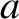, and simulated major radius. In accordance with the analysis of Chapter 3, suppose that the plasma is surrounded by a concentric, radially-thin, rigid, resistive wall of minor radius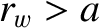. Likewise, suppose that the wall is surrounded by a concentric, radially-thin, magnetic field-coil, of minor radius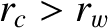, that carries a non-rotating (in the laboratory frame) helical current of amplitude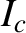. Let the current possess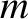periods in the poloidal direction, and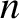periods in the toroidal direction. The static magnetic field generated by the field-coil constitutes our error-field. The error-field resonates with the plasma at the rational magnetic flux-surface, minor radius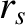, at which the safety-factor (see Section 3.2) takes the value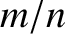.

Setting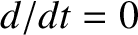(because the error-field is static) in Equations (3.187) and (3.188), we obtain(7.1)

Here,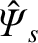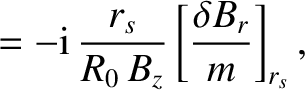(7.2)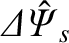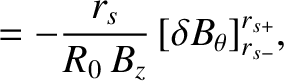(7.3)

where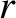,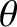,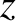are conventional cylindrical coordinates,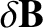the perturbed magnetic field, and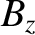the equilibrium toroidal magnetic field-strength. (See Sections 3.3, 3.8, and 3.15.) Clearly,is a measure of the reconnected magnetic flux driven at the rational surface by the error-field, whereasis a measure of the helical current sheet that is induced at the surface. Moreover,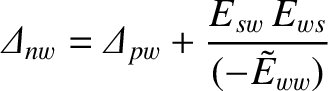(7.4)

is the (real dimensionless) tearing stability index (of a tearing mode with poloidal mode number, and toroidal mode number) in the absence of the wall,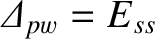(7.5)

the (real dimensionless) tearing stability index in the presence of a perfectly conducting wall at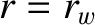(see Section 3.8), and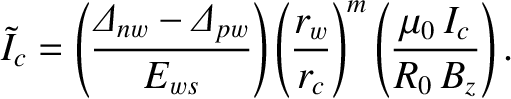(7.6)

Here, the (real dimensionless) plasma-wall coupling parameter,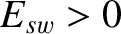, and the (real dimensionless) wall stability index,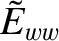, are defined in Sections 3.9 and 3.17, respectively. We expect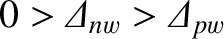, because the plasma is assumed to be tearing stable (see Chapter 6), and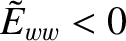. In fact, if we make the approximation that the equilibrium plasma current external to the rational magnetic flux-surface is negligible [i.e.,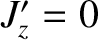in Equation (3.77)] then it is easily demonstrated that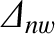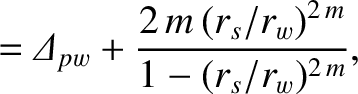(7.7)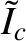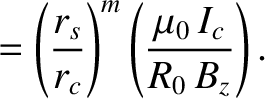(7.8)

In the radially-thin, resonant layer that surrounds the rational magnetic flux-surface, Equation (5.70) yields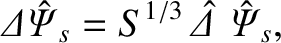(7.9)

where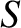is the the Lundquist number at the rational surface, and the complex layer matching parameter,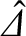, is defined in Equation (5.69). Hence, asymptotic matching between the inner and the outer regions (see Section 4.1) yields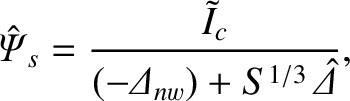(7.10)

where use has been made of Equations (7.1) and (7.9). The previous equation specifies the (normalized) reconnected magnetic flux,, driven at the rational surface by the (normalized) error-field coil current,. The complex layer parameter,, specifies the strength of a shielding current that is induced in the resonant layer, and acts to prevent driven magnetic reconnection. Note that the resistive wall has no influence onbecause no eddy currents are induced in the wall by a static error-field.